# 因式分解机

• 适用于极端稀疏的输入
• 线性的计算复杂度支持海量数据训练
• 适用范围广

# 前言

LR模型是CTR预估领域早期最成功的模型，大多工业推荐排序系统采取LR这种“线性模型+人工特征组合引入非线性”的模式。但是，LR模型最大的缺陷就是人工特征工程，耗时费力费人力资源，那么能否将特征组合的能力体现在模型层面呢？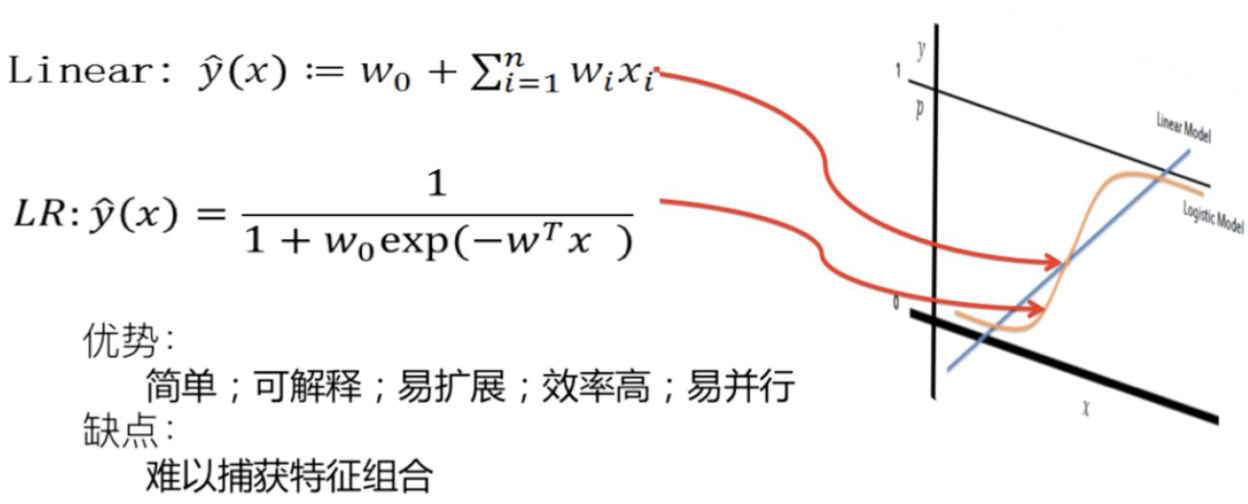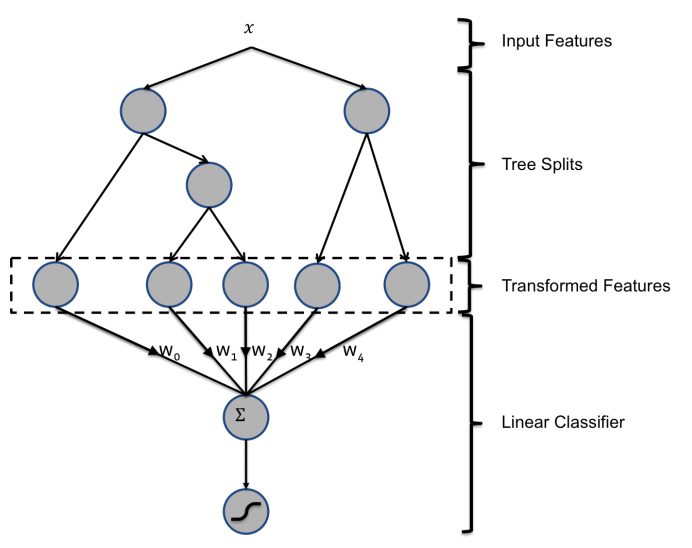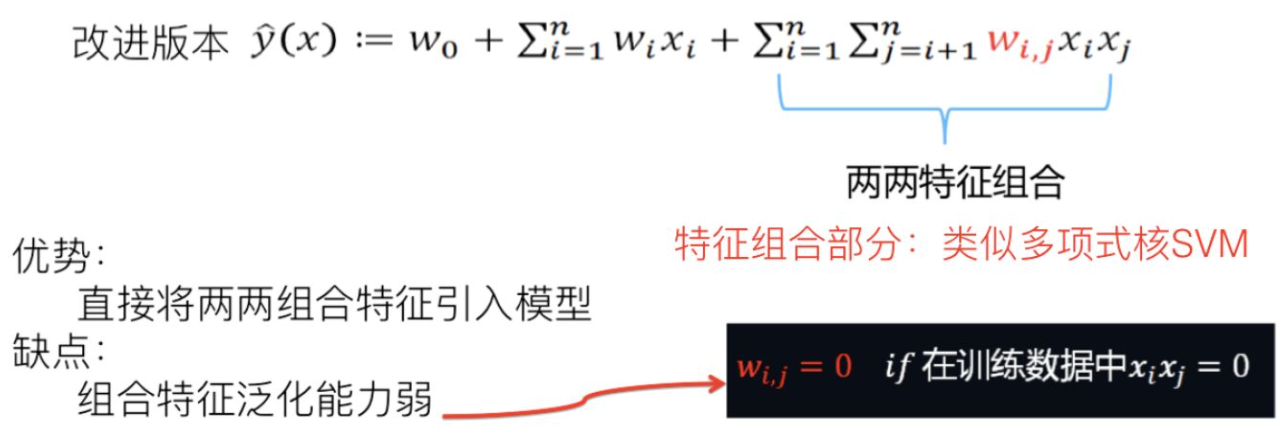• 核函数：$K(x, z)=<\phi(x), \phi(z)>=(+1)^{2}$
• \begin{aligned} \phi(x)=&\left(1, \sqrt{2} x_{1}, \cdots, \sqrt{2} x_{n}, x_{1}^{2}, \cdots, x_{n}^{2}\right.\\ &\left.\sqrt{2} x_{1} x_{2}, \cdots, \sqrt{2} x_{1} x_{n}, \sqrt{2} x_{2} x_{3}, \cdots, \sqrt{2} x_{n-1} x_{n}\right) \end{aligned}
• 输出：\begin{aligned} \hat{y}(x) &=\sum_{i=1}^{n} w_{i} \phi\left(x_{i}\right)+w_{0} \\ &=w_{0}+\sqrt{2} \sum_{i=1}^{n} w_{i} x_{i}+\sum_{i=1}^{n} w_{i i} x_{i}^{2}+\sqrt{2} \sum_{i=1}^{n-1} \sum_{j=i+1}^{n} w_{i j} x_{i} x_{i} \end{aligned}

SVM看上去解决了特征组合的问题，但是要知道推荐算法使用的特征是非常稀疏的，如果在训练数据里两个特征并未同时在训练实例里见到过，也就是说xi和xj一起出现的次数是0，那么SVM是无法学会这个特征组合的权重的。这就会导致模型的泛化性变差。

# FM基本原理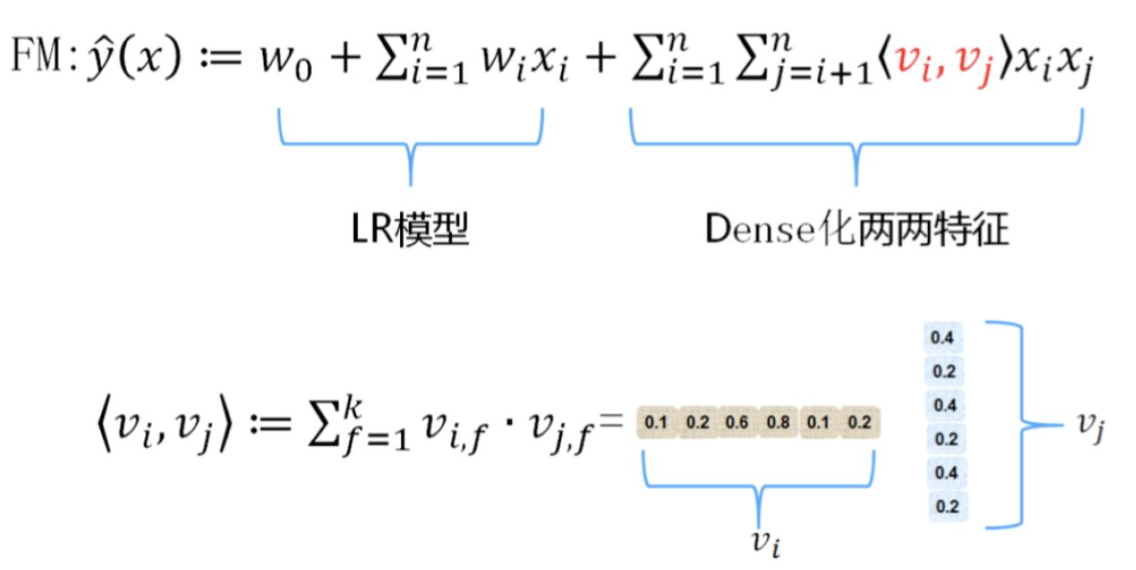### 增加上下文信息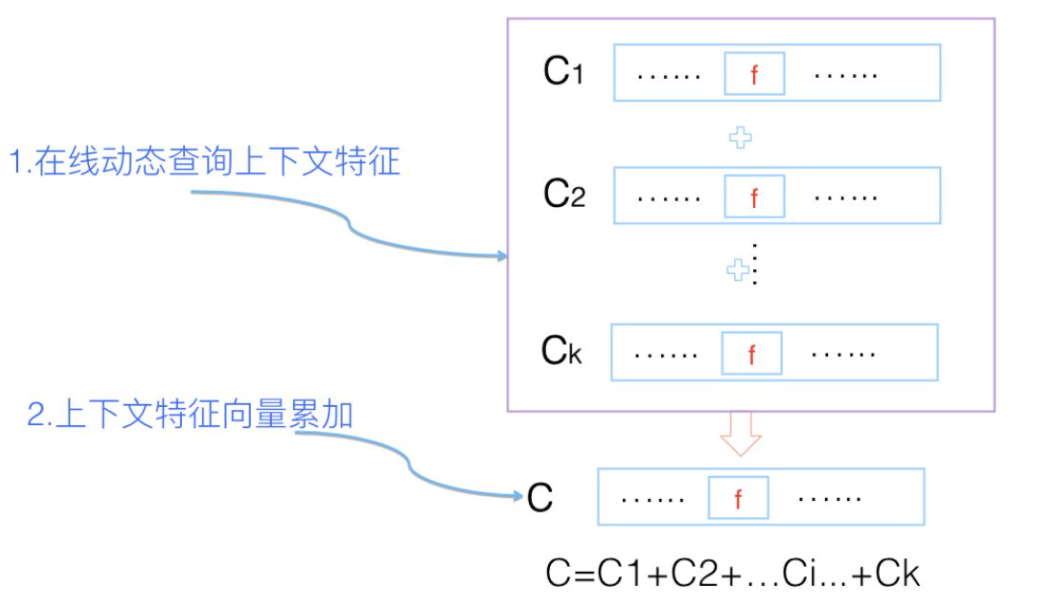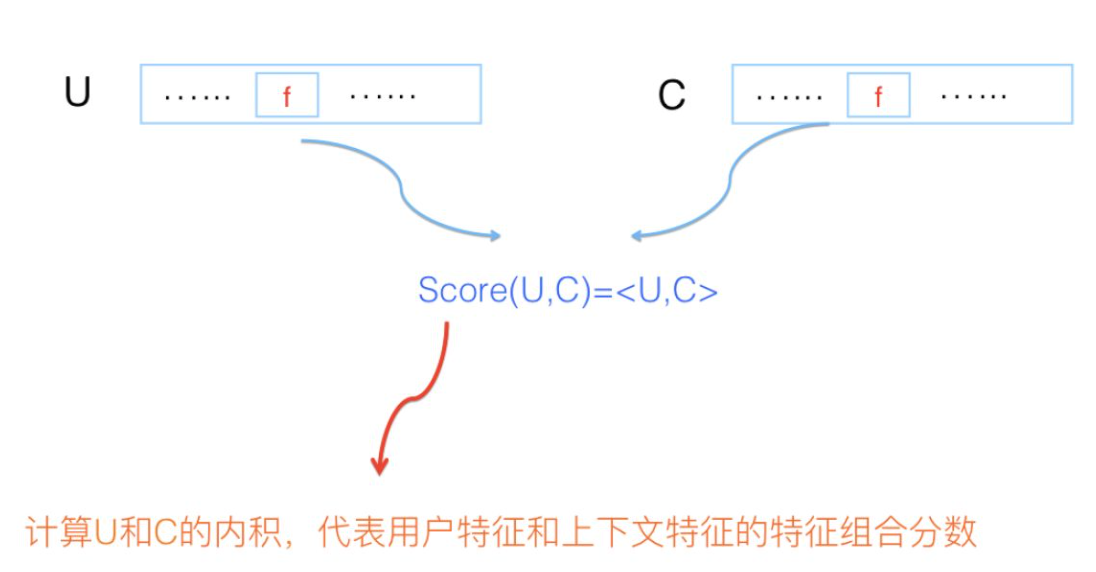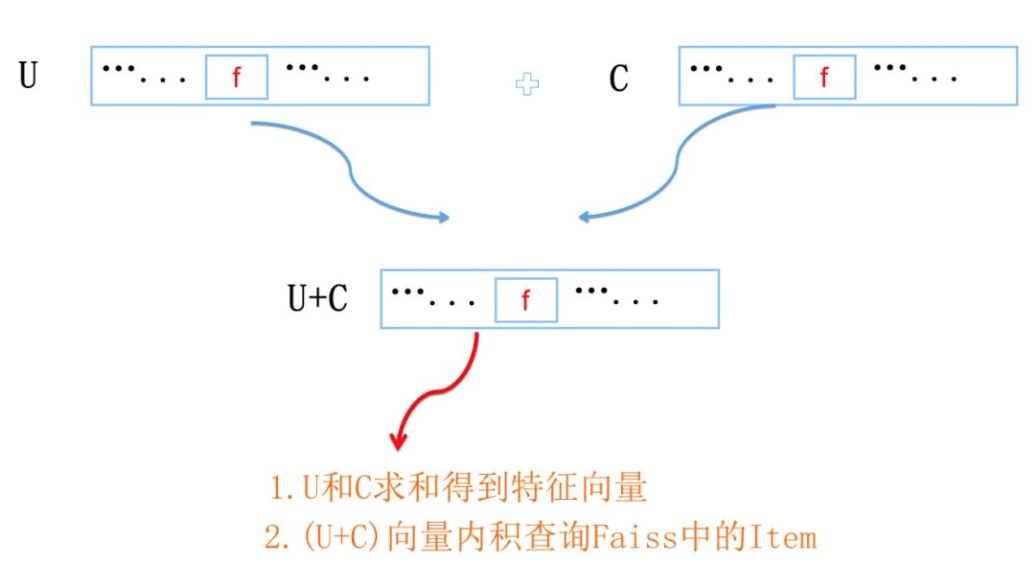## FM与多路召回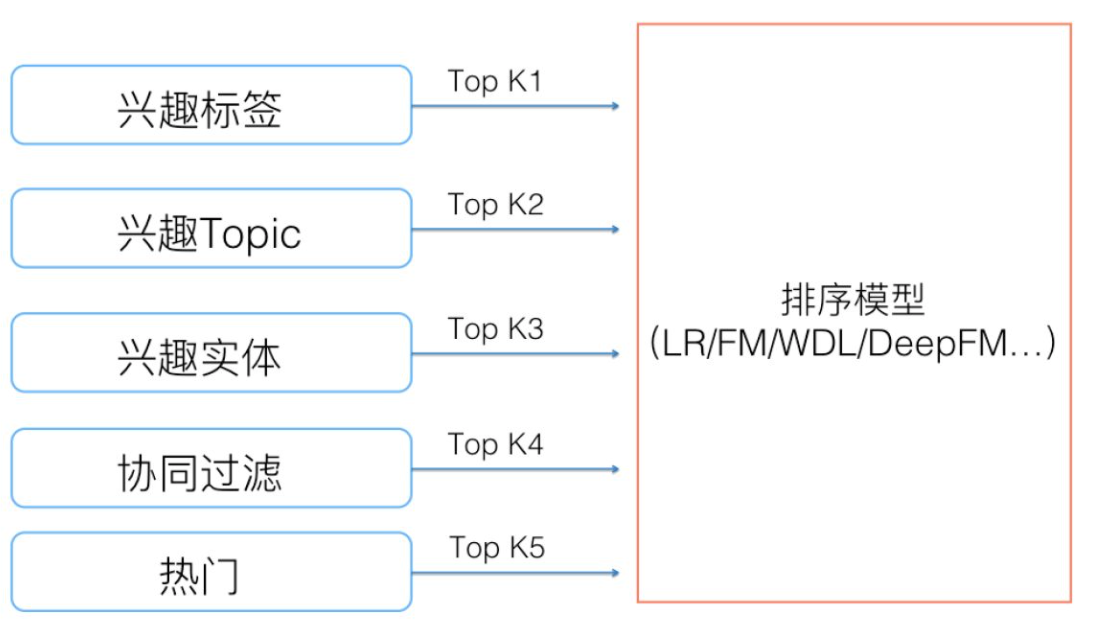• 不同路的item召回得分不可比
• 每一路召回的item个数k是个超参，需要不断调整
• 做recall和ranking的是两拨人，如果新增了一路召回和做ranking的人不知道，导致新增一路的召回特征没能加到ranking特征中，那么即便召回来了，也排不到前面去